# Short Run Xbar-R Charts

Short run Xbar and range (Xbar-R) charts can help you identify changes in the averages and range of averages of multiple characteristics—even those with different nominals, units of measure, or standard deviations—in limited production runs. Review the following example—an excerpt from Innovative Control Charting1—to get a sense of how a short run Xbar-R chart works.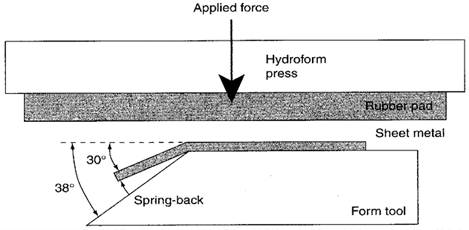Figure 1. Example of sheet metal spring-back after hydroform operation.

## Case Description

A hydroform is used to form angles in sheet metal. This is done by compressing a piece of sheer metal between a rubber pad and a form tool. When the metal is bent on the form tool, it springs back a few degrees when the pressure is released. To counteract the spring-back effect, the form tool angle exceeds the desired angle. In this case, the desired resultant sheet metal angles are 30°, 45°, and 90°. The average spring-back and standard deviations are different for each angle. The production foreman wants to use one control chart to monitor the spring-back behavior of all three types of angles. Table 1 shows the spring-back target values and specifications.

Table 1. Spring-back target values and specifications for three types of angles.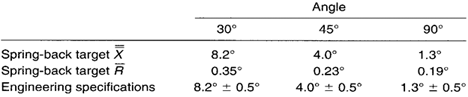Note: The target X values are based on engineering nominal values and the target R values are based on historical quality records.

## Sampling Strategy

The hydroform machine is initially set up to bend 45° angles. Five consecutive spring-back measurements are taken every hour until the job is complete. Next, the machine is set up to run 30° angles and so on. Sampling continues in the same manner as before. All measurements are plotted on the same short run Xbar-R chart.

## Data Collection Sheet

Table 2. Spring-back data including short run plot point calculations.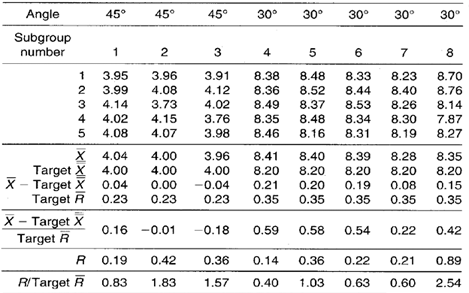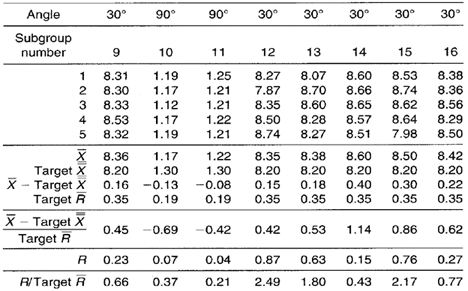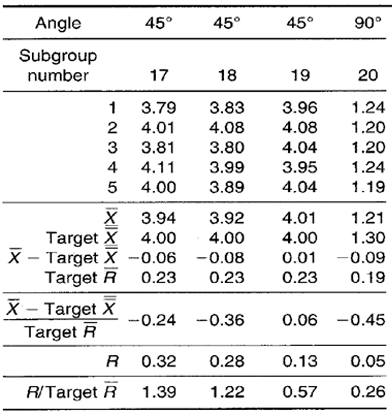## Short Run Xbar-R Chart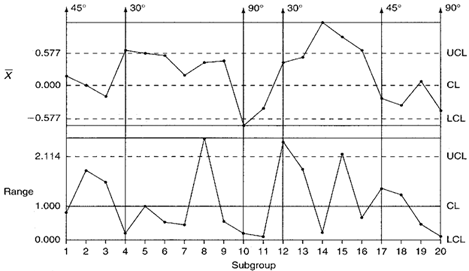Figure 2. Spring-back short run Xbar-R control charts.

## Chart Interpretation

Short run range chart: Three 30° plot points fall above the XJCL and are an indication that the variability for the 30° bends is greater than expected. The 45° plot points appear to be behaving randomly. The 90° plot points all fall below the centerline. Each pattern appears to be unique to each bend angle. There appear to be no visible patterns or trends that consistently appear across all bend angles collectively.

Short run Xbar chart: All 11 30° plot points fall above the centerline and five fall above the UCL. This indicates that the actual spring-back on 30° bends is greater than the established 8.2° target value. The 45° plot points appear to vary randomly about their target value.

The 90° plot points all fall below the centerline with one of them falling below the LCL. This indicates that the actual spring-back on 90° bends is less than the target X value of 1.3°. All plot point patterns appear unique to each bend angle. No trends are apparent across all bend angles collectively.

## Recommendations

### 30º Bend Angles

Range plot points erratically jumping above the UCL generally indicate unstable short-term variation. This might be caused by a process change that happens to occur within a subgroup. To pinpoint the cause, a 100-percent sampling strategy with a sample size of one may need to be temporarily established.
The average spring-back is consistently greater than the established target X of 8.2°. Investigate why the spring-back rates are so much larger than the engineering target and improve the process’ ability to maintain a lesser spring-back.

### 45º Bend Angles

Both ranges and averages appear to behave with consistent variability. The control chart reveals no specific process control issues that need to be addressed with respect to this bend angle.

### 90º Bend Angles

There are only three plot points on the short run chart that represent the 90° bend angles being produced (subgroups 10, 11, and 20). However, two of the three plot points on the short run Xbar chart are very close to the LCL and one falls below. If all three subgroups were consecutive, the two-out-of-three zone analysis rule would be triggered. The user of the control chart should try to find an obvious reason for the low bend angle values. If historical 90° bend angle data revealed points that were consistently stable about the center line on the control chart, then an investigation of recent process or raw material changes might be considered.

## Estimating the Process Average

Estimates of the process average should be calculated separately for each characteristic or part on short run Xbar-R charts. In this case, estimates of the process average should be calculated separately for each different spring-back angle. Calculation 1 shows the calculation for die overall average of the 30° spring-back measurements.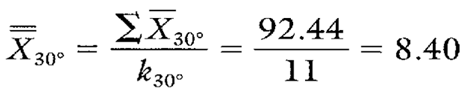Calculation 1. Estimate of the process average for 30° spring-back angles.

## Estimating Sigma

Estimates of sigma are also calculated separately for each characteristic or location represented on short run Xbar-R charts. In this case, estimates of the process standard deviation should be calculated for each different spring-back angle.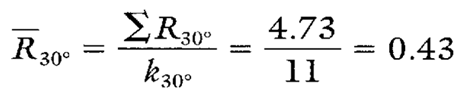Calculation 2. Calculation of the average moving range for 30° spring back-angles (to be used in estimating the standard deviation).Calculation 3. Estimate of the process standard deviation for the 30° spring-back angles.
Note: To ensure reliable estimates, k needs to be at least 20. In this example, k is only 11. Therefore, the estimates here and in Table 3 should be used only as references.

## Calculating Process Capability and Performance Ratios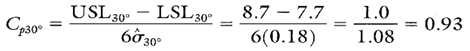Calculation 4. Cp calculation for the 30° bend angle spring-back.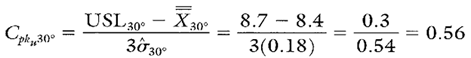Calculation 5. Cpk upper calculation for the 30° bend angle spring-back.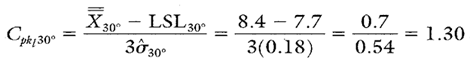Calculation 6. Cpk lower calculation for the 30° bend angle spring-back.

## Short Run Xbar-R Chart Advantages

• Graphically illustrates the variation of multiple product or process characteristics on the same chart.
• Characteristics from different parts with different means, different standard deviations, and different units of measure can be analyzed on the same chart.
• Pinpoints the characteristics that are most in need of attention.
• Separates variation due to changes in average from variation due to changes in the standard deviation.
• Separates process variation from product-specific variation.

## Short Run Xbar-R Chart Disadvantages

• The use of negative numbers and unitless ratios may be confusing at first.
• X, R, and the estimate of sigma must be calculated separately for each characteristic on the chart.
• Proper chart analysis requires knowledge of how target values were derived.

The process capability and performance ratio calculations for the 45° and 90° bend angle spring-back are shown in Table 17.7.

Table 3. Cp and Cpk calculations for 45° and 90° bend angle spring-back characteristics.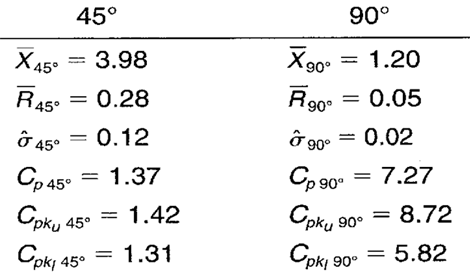When you use SPC software from InfinityQS, consuming the information provided by short run Xbar-R charts becomes faster and easier than ever. See how this type of analysis is surfaced in InfinityQS solutions.

FOOTNOTE:
1 Wise, Stephen A. and Douglas C. Fair. Innovative Control Charting: Practical SPC Solutions for Today’s Manufacturing Environment. Milwaukee, WI: ASQ Quality Press.

### Take the first step from quality to excellence

Take the Next Steps